Open in App
Not now

# Boxplot using Seaborn in Python

• Difficulty Level : Medium
• Last Updated : 10 Nov, 2020

Seaborn is an amazing visualization library for statistical graphics plotting in Python. It provides beautiful default styles and color palettes to make statistical plots more attractive. It is built on the top of matplotlib library and also closely integrated into the data structures from pandas.

## Box Plot

A box plot helps to maintain the distribution of quantitative data in such a way that it facilitates the comparisons between variables or across levels of a categorical variable. The main body of the box plot showing the quartiles and the median’s confidence intervals if enabled. The medians have horizontal lines at the median of each box and while whiskers have the vertical lines extending to the most extreme, non-outlier data points and caps are the horizontal lines at the ends of the whiskers.

Syntax: seaborn.boxplot(x=None, y=None, hue=None, data=None, order=None, hue_order=None, orient=None, color=None, palette=None, saturation=0.75, width=0.8, dodge=True, fliersize=5, linewidth=None, whis=1.5, ax=None, **kwargs)

Parameters:
x, y, hue: Inputs for plotting long-form data.
data: Dataset for plotting. If x and y are absent, this is interpreted as wide-form.
color: Color for all of the elements.

Returns: It returns the Axes object with the plot drawn onto it.

Example 1: Basic visualization of “fmri” dataset using violinplot()

## Python3

 `import` `seaborn`     `seaborn.``set``(style``=``'whitegrid'``)` `fmri ``=` `seaborn.load_dataset(``"fmri"``)`   `seaborn.boxplot(x``=``"timepoint"``,` `                ``y``=``"signal"``,` `                ``data``=``fmri)`

Output: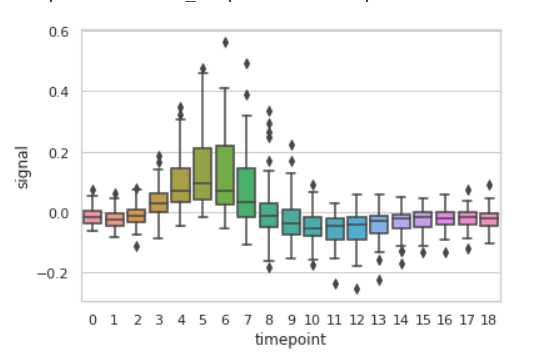Example 2: Basic visualization of “tips” dataset using boxplot()

## Python3

 `import` `seaborn`     `seaborn.``set``(style``=``'whitegrid'``)` `tip ``=` `seaborn.load_dataset(``'tips'``)`   `seaborn.boxplot(x``=``'day'``, y``=``'tip'``, data``=``tip)`

Output: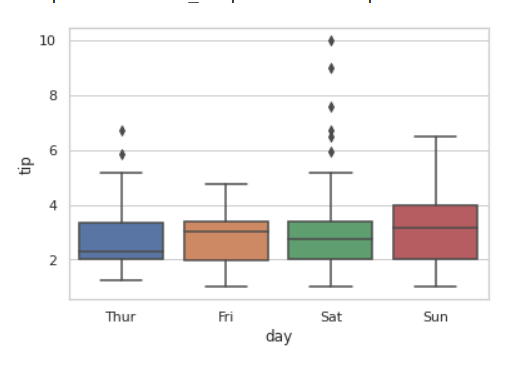## Grouping variables in Seaborn boxplot with different attributes

1. Draw a single horizontal box plot using only one axis:

If we use only one data variable instead of two data variables then it means that the axis denotes each of these data variables as an axis.

X denotes an x-axis and y denote a y-axis.

Syntax:

```seaborn.boxplot(x)
```

## Python3

 `# Python program to illustrate` `# boxplot using inbuilt data-set` `# given in seaborn` ` `  `# importing the required module` `import` `seaborn`   `# use to set style of background of plot` `seaborn.``set``(style``=``"whitegrid"``)`   `# loading data-set` `tip ``=` `seaborn.load_dataset(``"tips"``)`   `seaborn.boxplot(x ``=``tip[``'total_bill'``])`

Output: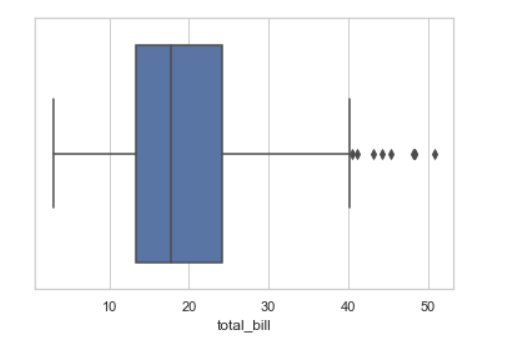2. Draw horizontal boxplot:

In the above example we see how to plot a single horizontal boxplot and here can perform multiple horizontal box plots with exchange of the data variable with another axis.

## Python3

 `# Python program to illustrate` `# boxplot using inbuilt data-set` `# given in seaborn` ` `  `# importing the required module` `import` `seaborn`   `# use to set style of background of plot` `seaborn.``set``(style``=``"whitegrid"``)`   `# loading data-set` `tip ``=` `seaborn.load_dataset(``"tips"``)` `seaborn.boxplot(x ``=``'tip'``, y ``=``'day'``, data ``=` `tip)`

Output: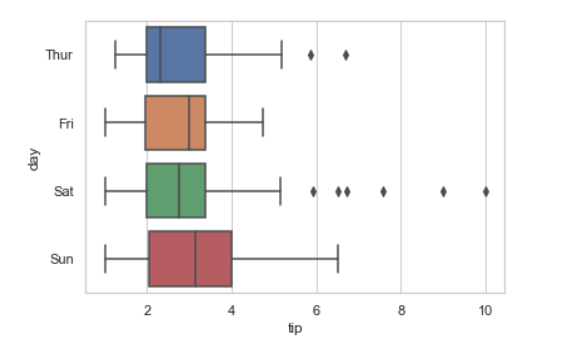3. Using hue parameter:

While the points are plotted in two dimensions, another dimension can be added to the plot by coloring the points according to a third variable.

Syntax:

seaborn.boxplot(x, y, hue, data);

## Python3

 `# Python program to illustrate` `# boxplot using inbuilt data-set` `# given in seaborn` ` `  `# importing the required module` `import` `seaborn`   `# use to set style of background of plot` `seaborn.``set``(style``=``"whitegrid"``)`   `# loading data-set` `tip ``=` `seaborn.load_dataset(``"fmri"``)`   `seaborn.boxplot(x ``=``"timepoint"``, ` `             ``y ``=``"signal"``, ` `             ``hue ``=``"region"``, ` `             ``data ``=` `fmri)`

Output: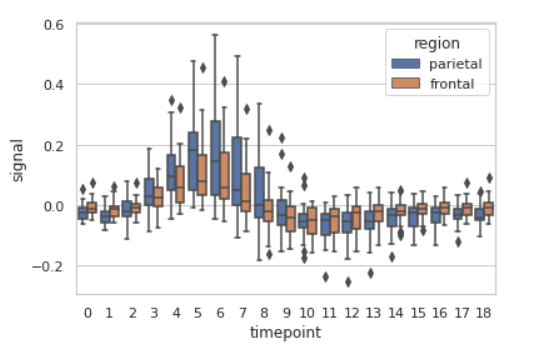4. Draw outlines around the data points using linewidth:

Width of the gray lines that frame the plot elements. Whenever we increase linewidth than the point also will increase automatically.

Syntax:

seaborn.boxplot(x, y, data, linewidth)

## Python3

 `# Python program to illustrate` `# boxplot using inbuilt data-set` `# given in seaborn` ` `  `# importing the required module` `import` `seaborn`   `# use to set style of background of plot` `seaborn.``set``(style``=``"whitegrid"``)`   `# loading data-set` `tip ``=` `seaborn.load_dataset(``"tips"``)` `seaborn.boxplot(x ``=` `'day'``, y ``=` `'tip'``, ` `                ``data ``=` `tip,` `                ``linewidth``=``2.5``)`

Output: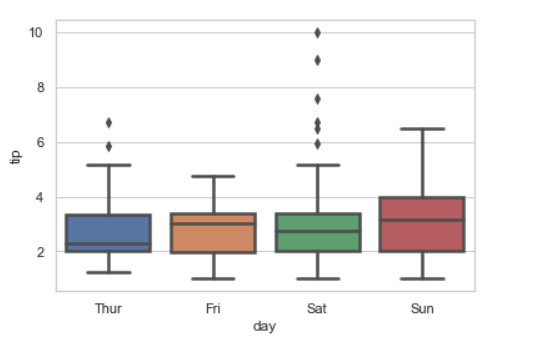5. Draw each level of the hue variable at different locations on the major categorical axis:

When using hue nesting, setting dodge should be True will separate the point for different hue levels along the categorical axis. And Palette is used for the different levels of the hue variable.

Syntax:

seaborn.boxplot(x, y, data, hue, palette, dodge)

## Python3

 `# Python program to illustrate` `# boxplot using inbuilt data-set` `# given in seaborn` ` `  `# importing the required module` `import` `seaborn`   `# use to set style of background of plot` `seaborn.``set``(style``=``"whitegrid"``)`   `# loading data-set` `tip ``=` `seaborn.load_dataset(``"tips"``)` `seaborn.boxplot(x``=``"day"``, y``=``"total_bill"``, ` `                ``hue``=``"smoker"``,` `                ``data``=``tips, palette``=``"Set2"``,` `                ``dodge``=``True``)`

Output: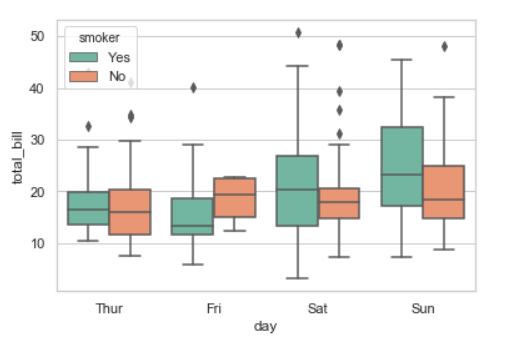Possible values of palette are:

Accent, Accent_r, Blues, Blues_r, BrBG, BrBG_r, BuGn, BuGn_r, BuPu, BuPu_r, CMRmap, CMRmap_r, Dark2, Dark2_r,

GnBu, GnBu_r, Greens, Greens_r, Greys, Greys_r, OrRd, OrRd_r, Oranges, Oranges_r, PRGn, PRGn_r, Paired, Paired_r,

Pastel1, Pastel1_r, Pastel2, Pastel2_r, PiYG, PiYG_r, PuBu, PuBuGn, PuBuGn_r, PuBu_r, PuOr, PuOr_r, PuRd, PuRd_r,

Purples, Purples_r, RdBu, RdBu_r, RdGy, RdGy_r, RdPu, RdPu_r, RdYlBu, RdYlBu_r, RdYlGn, RdYlGn_r, Reds, Reds_r, Set1,

Set1_r, Set2, Set2_r, Set3, Set3_r, Spectral, Spectral_r, Wistia, Wistia_r, YlGn, YlGnBu, YlGnBu_r, YlGn_r, YlOrBr,

YlOrBr_r, YlOrRd, YlOrRd_r, afmhot, afmhot_r, autumn, autumn_r, binary, binary_r, bone, bone_r, brg, brg_r, bwr, bwr_r,

cividis, cividis_r, cool, cool_r, coolwarm, coolwarm_r, copper, copper_r, cubehelix, cubehelix_r, flag, flag_r, gist_earth,

gist_earth_r, gist_gray, gist_gray_r, gist_heat, gist_heat_r, gist_ncar, gist_ncar_r, gist_rainbow, gist_rainbow_r, gist_stern,

6. Control orientation of the plot (vertical or horizontal):

When we use orient as “h” then it plots the vertical and if we use “V” then it refers to the vertical.

Syntax:

seaborn.boxplot( data, orient )

## Python3

 `# Python program to illustrate` `# boxplot using inbuilt data-set` `# given in seaborn` ` `  `# importing the required module` `import` `seaborn`   `# use to set style of background of plot` `seaborn.``set``(style``=``"whitegrid"``)`   `# loading data-set` `tip ``=` `seaborn.load_dataset(``"tips"``)` `seaborn.boxplot(data ``=` `tip,orient``=``"h"``)`

Output: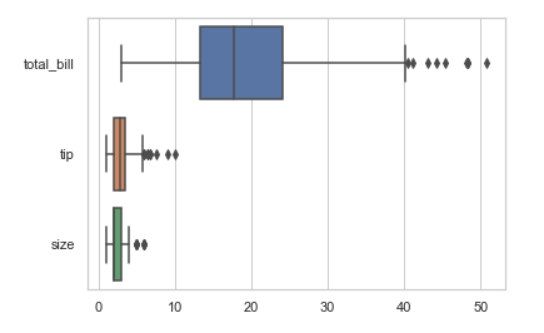Let’s check for vertical orient:

## Python3

 `seaborn.boxplot(data ``=` `tip,orient``=``"v"``)`

Output: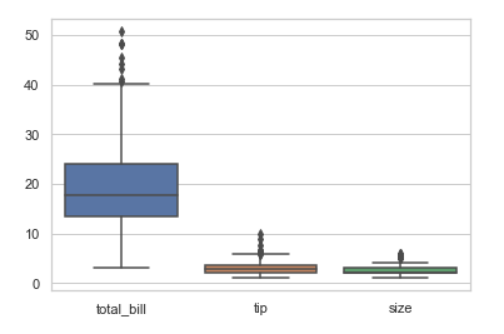7. Using color attributes for Color for all the elements.:

## Python3

 `# Python program to illustrate` `# boxplot using inbuilt data-set` `# given in seaborn` ` `  `# importing the required module` `import` `seaborn`   `# use to set style of background of plot` `seaborn.``set``(style``=``"whitegrid"``)`   `# loading data-set` `tip ``=` `seaborn.load_dataset(``"tips"``)` `seaborn.boxplot(x ``=` `'day'``, y ``=` `'tip'``, data ``=` `tip,color ``=` `"green"``)`

Output: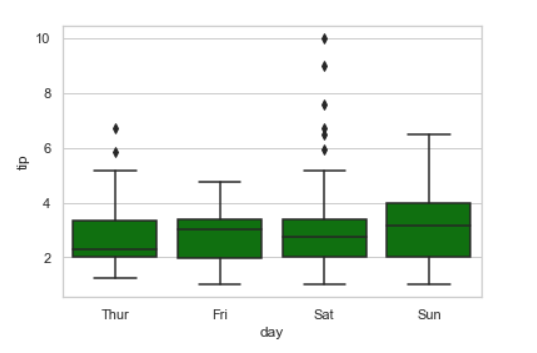My Personal Notes arrow_drop_up
Related Articles